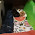# Write VBA in SAS

This tutorial explains how we can write and run Visual Basic for Application (VBA) code from SAS. VBA is a programming language for automating repetitive tasks in MS Excel and other MS Office applications. It is one of the powerful language for analysing data and reporting numbers. VBA is mainly used to create macros in Excel. By integrating excel with SAS, you can format the excel report and create pivot table from SAS. It would make the automation process to next level and increase operational efficiency of a project.

Write VBA (VB Script) in SAS : Examples

Example 1 : Create a new workbook and type in a cell

The following SAS program opens a sample excel workbook and type 'Welcome to Excel' in cell A1. The translated VB Script would be stored in the temp location by the name 'vbscript'. Type %put vba_loc; to see the location of the code.
options noxwait noxsync;
%let vba_loc=%sysfunc(getoption(WORK))\vbscript.vbs;
data _null_;
file "&vba_loc";
put "Set objExcel = CreateObject(""Excel.Application"")  ";
put "objExcel.Visible = True ";
put "Set wb = objExcel.Workbooks.Add";
put "wb.Activesheet.Range(""A1"").Value=""Welcome to Excel""";
x "'&vba_loc\'";
run;
Note :

1. options noxwait noxsync
These options would tell SAS to automatically close command prompt window and the SAS System does not wait for the application to finish.

2. %let vba_loc
It is the location where visual basic script is stored. Open the file in notepad to see the code.VB Script in SAS
3. Type each line of VBA code in put statement and make sure adding extra double quote whenever you use double quote in VBA.

Example 2 : Enter a Formula in Excel from SAS

The following SAS program opens a workbook and enter a formula in cell B2 and sum the range A2:A6 and later saves the workbook.
options noxwait noxsync;
%let vba_loc=%sysfunc(getoption(WORK))\vbscript.vbs;
%let open_workbook = C:\Users\Deepanshu\Documents\Book1.xlsx;
data _null_;
file "&vba_loc";
put "Set objExcel = CreateObject(""Excel.Application"")  ";
put "objExcel.Visible = True ";
put "Set wb = objExcel.Workbooks.Open(""&open_workbook"")";
put "wb.sheets(""Sheet1"").Range(""B2"").Formula = ""=SUM(A2:A6)""";
put "wb.save";
x "'&vba_loc\'";
run;

Example 3 : Insert an Image in Excel from SAS
options noxwait noxsync;
%let vba_loc=%sysfunc(getoption(WORK))\vbscript.vbs;
%let open_workbook = C:\Users\Deepanshu\Documents\Book1.xlsx;
data _null_;
file "&vba_loc";
put "Set objExcel = CreateObject(""Excel.Application"")  ";
put "objExcel.Visible = True ";
put "Set wb = objExcel.Workbooks.Open(""&open_workbook"")";
put "Set Xlsheet = wb.Worksheets(""Sheet1"")";
put "Xlsheet.Pictures.Insert(""C:\Users\Public\Pictures\Sample Pictures\Desert.jpg"")";
put "wb.save";
x "'&vba_loc\'";
run;

Example 4 : Apply Filter, Make Headers Bold and Freeze Panes from SAS

Step 1 : Export Sample Data to Excel and use it further to format it from SAS.
proc export data = sashelp.prdsale outfile= "C:\Users\Deepanshu\Documents\Example.xlsx";
run;
Step 2 : Formatting in Excel from SAS
options noxwait noxsync;
%let vba_loc=%sysfunc(getoption(WORK))\vbscript.vbs;
%let open_workbook = C:\Users\Deepanshu\Documents\Example.xlsx;
data _null_;
file "&vba_loc";
put "Set objExcel = CreateObject(""Excel.Application"")  ";
put "objExcel.Visible = True ";
put "Set wb = objExcel.Workbooks.Open(""&open_workbook"")";
put "Set xl = wb.Worksheets(1)";
put "xl.Activate";
put "With wb.ActiveSheet";
put ".Rows(1).Font.Bold = True";
put ".AutoFilterMode = False";
put ".Rows(1).AutoFilter";
put ".Columns.AutoFit";
put ".Range(""A2"").Select";
put "End With";
put "objExcel.ActiveWindow.FreezePanes = True";
put "wb.save";
x "'&vba_loc\'";
run;
Example 5 : Apply Pivot Table in Excel from SAS

The following program tells SAS to apply pivot table in Excel from SAS.
%macro SAS_VBA (open_workbook=, sheet=, rowlabels=, columnlabels= ,evalfieldss=, stat=);
%let script_loc=%sysfunc(getoption(WORK))\vbscript.vbs;
%let sheetname = PivotTable;
data _null_;
file "&script_loc";
put "Set objExcel = CreateObject(""Excel.Application"")  ";
put "objExcel.Visible = True ";
put "Set wb = objExcel.Workbooks.Open(""&open_workbook"")";
put "Set Xlsheet = wb.Worksheets(""&sheet"")";
put "Xlsheet.Select";
put "lastcell = objExcel.cells.specialcells(11).address";
put "wb.Activesheet.name = ""&sheetname""";
put "wb.Sheets(""&sheetname"").select";
put "wb.ActiveSheet.PivotTableWizard SourceType=xlDatabase, wb.sheets(""&sheet"").Range(""A1"" & "":"" & lastcell),""&sheetname!R1C1"",""pvttbl""";

/* Loop through the list of row fields and set them in the pivot table */
%let i=0;
%do %while(%scan(&rowlabels,&i+1,%str( )) ne %str( ));
%let i = %eval(&i+1);
%let var = %scan(&rowlabels,&i,%str( ));
put "wb.ActiveSheet.PivotTables(""pvttbl"").PivotFields(""&var"").Orientation =""1""" ;
%end;

%let i=0;
/* Loop through the list of column fields and set them in the pivot table */
%do %while(%scan(&columnlabels,&i+1,%str( )) ne %str( ));
%let i = %eval(&i+1);
%let var = %scan(&columnlabels,&i,%str( ));
put "wb.ActiveSheet.PivotTables(""pvttbl"").PivotFields(""&var"").Orientation =""2""" ;
%end;

/* Loop through the list of data fields and set them in the pivot table */
%let i=0;
%do %while(%scan(&evalfieldss,&i+1,%str( )) ne %str( ));
%let i = %eval(&i+1);
%let var = %scan(&evalfieldss,&i,%str( ));
%let istat = %scan(&stat,&i,%str( ));
wb.ActiveSheet.PivotTables(""pvttbl"").PivotFields(""&var""),
""&istat of &var"","
%IF %UPCASE(&istat) EQ SUM %THEN "-4157";
%IF %UPCASE(&istat) EQ COUNT %THEN "-4112";
%IF %UPCASE(&istat) EQ AVERAGE %THEN "-4106";;
%end;

/* Hide Field List*/
put "objExcel.ActiveWorkbook.ShowPivotTableFieldList = False";
put "wb.save";
x "'&script_loc\'";
run;
%mend;

%SAS_VBA (open_workbook= C:\Users\Deepanshu\Documents\example.xlsx, sheet = PRDSALE, rowlabels= COUNTRY,
columnlabels = DIVISION, evalfieldss= Actual Predict, stat= sum sum);
In the above macro, parameter "sheet" refers to the sheet wherein data is stored.Related PostsDeepanshu founded ListenData with a simple objective - Make analytics easy to understand and follow. He has over 8 years of experience in data science. During his tenure, he has worked with global clients in various domains like Banking, Insurance, Telecom and Human Resource.

3 Responses to "Write VBA in SAS"
1.Hi, Thanks for the Article. I am facing some issue while executing the code using SAS. The SAS log is showing the error as "Unrecognized SAS option name NOXWAIT" and "Unrecognized SAS option name NOXSYNC".

The code I'm trying to run is

options symbolgen;

options noxwait noxsync;

%let vba_loc = /home/mmacherla/openexcel.vbs;

%let open_workbook = /home/mmacherla/Try.xlsx;

data _null_;

file "&vba_loc";
put "Set objExcel = CreateObject(""Excel.Application"")";
put "objExcel.Visible = True";
put "objExcel.DisplayAlerts = False";
put "Set wb = objExcel.Workbooks.Open(""&open_workbook"")";
put "wb.sheets(""Sheet1"").Range(""A1"").Value = ""100""";
put "wb.save";
x ""&vba_loc\"";

run;

I am trying to run this SAS code through putty.

2.How do you include the closing of Excel in this?

1.This comment has been removed by the author.

Next → ← Prev
Love this Post? Spread the Word!
Share